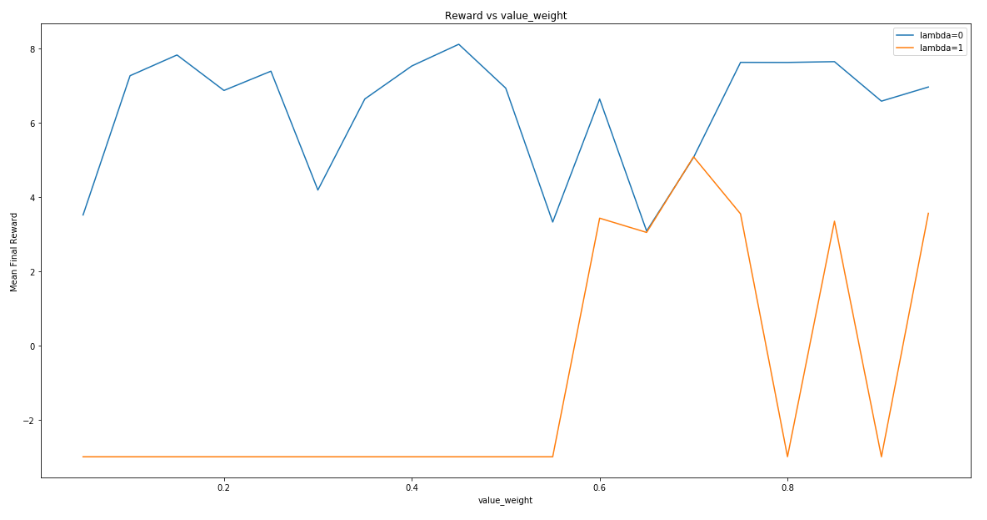Pull Request

DeepChem now has an implementation of A3C as part of a push for working with reinforcement learning problems. I decided to take it for a spin in what I thought was an easy problem Tic-tac-toe. That is how well an agent can do against a random player making legal moves. It turns out the problem was not quite trivial.

After playing around with many network architectures and hyper-parameters I was never able to get an agent to win as much as I would like. Even more so the agent was almost entirely unable to learn the rules of the game. The agent repeatedly made illegal moves (moving where there was already a token).

After reading through DeepChem’s implementation I thought I found a bug with it’s implementation of Advantage. I compared it to the paper, and a blog post, both of which had a different implementation of advantage. The A3C paper briefly discusses both equations but uses $$\lambda = 1$$ for its implementation

$(\lambda = 0) | V(s_i) = r_i + V(s_{(i+1)}) \\ (\lambda = 1) | V(s_i) = r_i + r_{(i+1)} + r_{(i+2)} + ...$

After substituting the $$\lambda = 1$$ equation we started getting much better results in Tic-tac-toe.It turns out the two different equations are special cases of Generalized Advantage Estimation. Michael Jordan and Pieter Abbeel discuss the tradeoffs between the two forms of advantage and benchmark results for various RL problems. Their results do not agree with mine, the paper recommends $$\lambda$$ values close to 1.

$$\lambda = 1$$ is unbiased, but has a high variance since it’s a sum of many terms. $$\lambda = 0$$ has much lower variance, since it only depends on one step, but it assumes you already know how to calculate the value function for the next step. But that’s the quantity we’re trying to learn, so all we can do is use our current estimate of it, and that introduces bias. The purpose of lambda is to interpolate between these two approximations.

So in summary, surprise! Reinforcement learning is finicky, and small changes in hyper parameters can have large non intuitive impacts. The implementation in DeepChem is still the $$\lambda = 1$$ equation for advantage, but we would love a contribution to make it a hyper-parameter of our A3C implementation